# Class 12 Maths NCERT Solutions for Chapter 13 Probability Exercise 13.3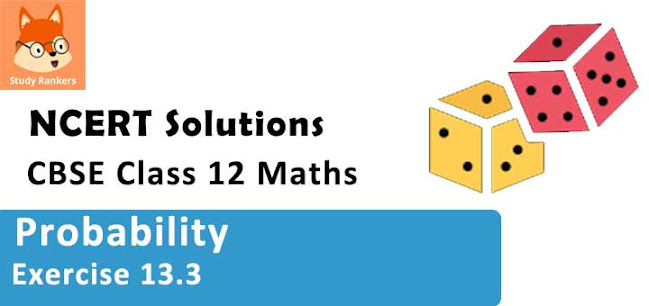### Probability Exercise 13.3 Solutions

1. An urn contains 5 red and 5 black balls. A ball is drawn at random, its colour is noted and is returned to the urn. Moreover, 2 additional balls of the colour drawn are put in the urn and then a ball is drawn at random. What is the probability that the second ball is red?

Solution

The urn contains 5 red and 5 black balls.
Let a red ball be drawn in the first attempt.
P (drawing a red ball) = 5/10 = 1/2
If two red balls are added to the urn, then the urn contains 7 red and 5 black balls.
P (drawing a red ball) = 7/12
Let a black ball be drawn in the first attempt.
P (drawing a black ball in the first attempt) = 5/10 = 1/2
If two black balls are added to the urn, then the urn contains 5 red and 7 black balls.
P (drawing a red ball) = 5/12
Therefore, probability of drawing second ball as red is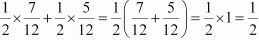2. A bag contains 4 red and 4 black balls, another bag contains 2 red and 6 black balls. One of the two bags is selected at random and a ball is drawn from the bag which is found to be red. Find the probability that the ball is drawn from the first bag.

Solution

Let E1 and E2 be the events of selecting first bag and second bag respectively.
∴ P(E1) = P(E2) = 1/2
Let A be the event of getting a red ball.
⇒ P(A|E1) = P(drawing a red ball from first bag) = 4/8 = 1/2
⇒ P(A|E2 ) = P (drawing a red ball from second bag) = 2/8 = 1/4
The probability of drawing a ball from the first bag, given that is is red, is given by P (E2|A) .
By using Bayes' theorem, we obtain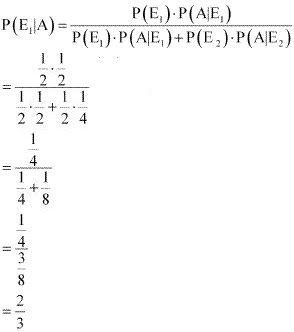3. Of the students in a college, it is known that 60% reside in hostel and 40% are day scholars (not residing in hostel). Previous year results report that 30% of all students who reside in hostel attain A grade and 20% of day scholars attain A grade in their annual examination. At the end of the year, one student is chosen at random from the college and he has an A grade, what is the probability that the student is hostler?

Solution

Let E1 and E2 be the events that the student is a hostler and a day scholar respectively and A be the event that the chosen student gets grade A.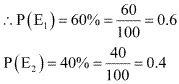P(A|E1) = P(student getting an A grade is a hostler) = 30% = 0.3
P(A|E2) = P(student getting an A grade is a day scholar) = 20% = 0.2
The probability that a randomly chosen student is a hostler, given that he has an A grade, is given by P (E1 |A) .
By using Bayes' theorem, we obtain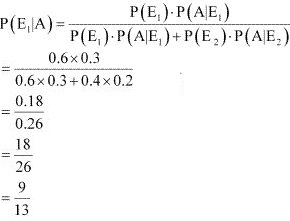4. In answering a question on a multiple choice test, a student either knows the answer or guesses. Let 3/4 be the probability that he knows the answer and 1/4 be the probability that he guesses. Assuming that a student who guesses at the answer will be correct with probability 1/4 What is the probability that the student knows the answer given that he answered it correctly?

Solution

Let E1 and E2 be the respective events that the student knows the answer and he guesses the answer.
Let A be the event that the answer is correct.
∴ P(E1) =  3/4
P(E2 ) = 1/4
The probability that the student answered correctly, given that he knows the answer , is 1.
∴ P (A|E1 )  = 1
Probability that the student answered correctly, given that he guessed, is 1/4.
∴ P (A|E2 ) = 1/4
The probability that the student knows the answer, given that he answered it correctly, is given by P (E1 |A).
By using Bayes' theorem, we obtain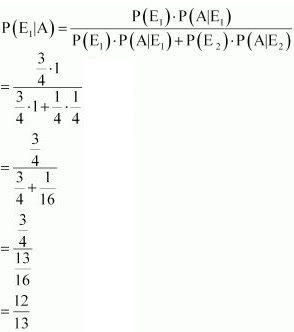5. A laboratory blood test is 99% effective in detecting a certain disease when it is in fact, present. However, the test also yields a false positive result for 0.5% of the healthy person tested (that is, if a healthy person is tested, then, with probability 0.005, the test will imply he has the disease). If 0.1 percent of the population actually has the disease, what is the probability that a person has the disease given that his test result is positive?
Solution

Let E1 and E2 be the respective events that a person has a disease and a person has no disease.
Since E1 and E2 are events complimentary to each other,
∴ P (E1) + P (E2) = 1
⇒ P (E2) = 1 − P (E1) = 1 − 0.001 = 0.999
Let A be the event that the blood test result is positive.
P(E1) = 0.1% = 0.1/100 = 0.001
P(A|E1) = P(result is positive given the person has disease) = 90% = 0.99 P(A|E2) = P(result is positive given that the person has no disease) = 0.5% = 0.005
Probability that a person has a disease, given that his test result is positive, is given by
P (E1|A).
By using Bayes’ theorem, we obtain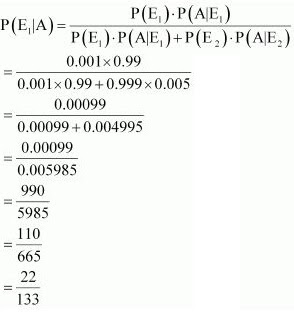6. There are three coins. One is two headed coin (having head on both faces), another is a biased coin that comes up heads 75% of the time and third is an unbiased coin. One of the three coins is chosen at random and tossed, it shows heads, what is the probability that it was the two headed coin?

Solution

Let E1, E2, and E3 be the respective events of choosing a two headed coin, a biased coin, and an unbiased coin.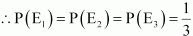Let A be the event that the coin shows heads.
∴ P (A|E1 )  = P(coin showing heads, given that it is a two - headed coin) = 1
Probability of heads coming up, given that it is a two - headed coin) = 1
Probability of heads coming up, given that it is a biased coin = 75%
∴ P (A|E2)  = P(coin showing heads, given that it is biased coin) = 75/100 = 3/4
Since the third coin is unbiased, the probability that it shows heads is always 1/2.
∴ P (A|E3)  = P(coin showing heads, given that it is biased coin) = 1/2
The probability that the coin is two - headed, given that it shows heads, is given by
P (E1 |A).
By using Bayes' theorem, we obtain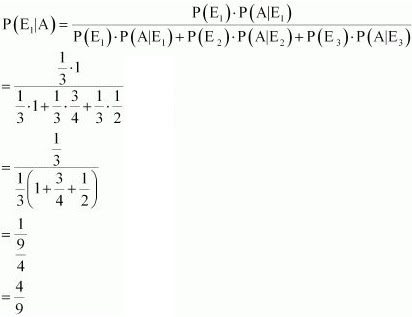7. An insurance company insured 2000 scooter drivers, 4000 car drivers and 6000 truck drivers. The probability of accidents are 0.01, 0.03 and 0.15 respectively. One of the insured persons meets with an accident. What is the probability that he is a scooter driver?

Solution

Let E1, E2, and E3 be the respective events that the driver is a scooter driver, a car driver, and a truck driver.
Let A be the event that the person meets with an accident.
There are 2000 scooter drivers, 4000 car drivers, and 6000 truck drivers.
Total number of drivers = 2000 + 4000 + 6000 = 12000
P (E1) = P (driver is a scooter driver) = 2000/12000 = 1/6
P (E2) = P (driver is a car driver) = 4000/12000 = 1/3
P (E3) = P (driver is a truck driver) = 6000/12000 = 1/2
P (A|E1)= P(scooter driver met with an accident) = 0.01 = 1/100
P (A|E2)= P (car driver met with an accident) = 0.03 = 3/100
P (A|E3)= P (truck driver met with an accident) = 0.15 = 15/100
The probability that the driver is a scooter driver, given that he met with an accident, is given by P (E1 |A).
By using Bayes' theorem, we obtain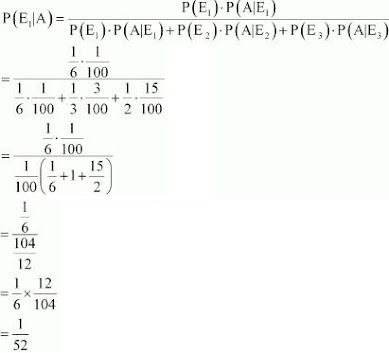8. A factory has two machines A and B. Past record shows that machine A produced 60% of the items of output and machine B produced 40% of the items. Further, 2% of the items produced by machine A and 1% produced by machine B were defective. All the items are put into one stockpile and then one item is chosen at random from this and is found to be defective. What is the probability that was produced by machine B?

Solution

Let E1 and E2 be the respective events of items produced by machines A and B. Let X be the event that the produced item was found to be defective.
∴ Probability of items produced by machine A, P (E1) = 60% = 3/5
Probability of items produced by machine B, P (E2) = 40% = 2/5
Probability that machine A produced defective items, P (X|E1) = 2% = 2/100
Probability that machine B produced defective items, P (X|E2) = 1% = 1/100
The probability that the randomly selected item was from machine B, given that it is defective, is given by P (E2|X).
By using Bayes’ theorem, we obtain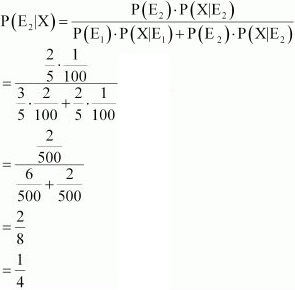9. Two groups are competing for the position on the board of directors of a corporation. The probabilities that the first and the second groups will win are 0.6 and 0.4 respectively. Further, if the first group wins, the probability of introducing a new product is 0.7 and the corresponding probability is 0.3 if the second group wins. Find the probability that the new product introduced was by the second group.

Solution

Let E1 and E2 be the respective events that the first group and the second group win the competition. Let A be the event of introducing a new product.
P (E1) = Probability that the first group wins the competition = 0.6
P (E2) = Probability that the second group wins the competition = 0.4
P (A|E1) = Probability of introducing a new product if the first group wins = 0.7
P (A|E2) = Probability of introducing a new product if the second group wins = 0.3
The probability that the new product is introduced by the second group is given by
P (E2|A).
By using Bayes’ theorem, we obtain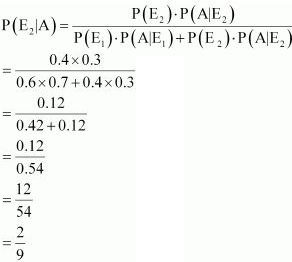10. Suppose a girl throws a die. If she gets a 5 or 6, she tosses a coin three times and notes the number of heads. If she gets 1, 2, 3 or 4, she tosses a coin once and notes whether a head or tail is obtained. If she obtained exactly one head, what is the probability that she threw 1, 2, 3 or 4 with the die?

Solution

Let E1 be the event that the outcome on the die is 5 or 6 and E2 be the event that the outcome on the die is 1, 2, 3, or 4.
∴ P(E1) = 2/6 = 1/3 and P(E2) = 4/6 = 2/3
Let A be the event of getting exactly one head.
P (A|E1) = Probability of getting exactly one head by tossing the coin three times if she gets 5 or 6 = 3/8
P (A|E2) = Probability of getting exactly one head in a single throw of coin if she gets 1, 2, 3, or 4 = 1/2
The probability that the girl threw 1, 2, 3, or 4 with the die, if she obtained exactly one head, is given by P (E2|A).
By using Bayes’ theorem, we obtain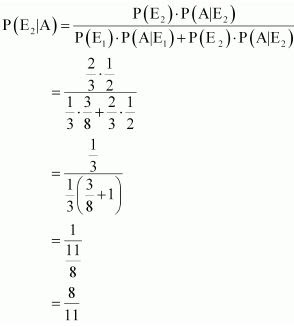11. A manufacturer has three machine operators A, B and C. The first operator A produces 1% defective items, where as the other two operators B and C produce 5% and 7% defective items respectively. A is on the job for 50% of the time, B is on the job for 30% of the time and C is on the job for 20% of the time. A defective item is produced, what is the probability that was produced by A?

Solution

Let E1, E2, and E3 be the respective events of the time consumed by machines A, B, and C for the job.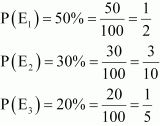Let X be the event of producing defective items.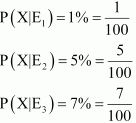The probability that the defective item was produced by A is given by P (E1|A).
By using Bayes' theorem, we obtain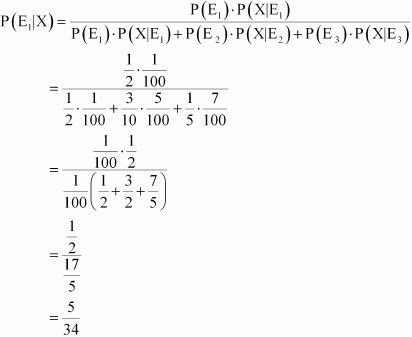12. A card from a pack of 52 cards is lost. From the remaining cards of the pack, two cards are drawn and are found to be both diamonds. Find the probability of the lost card being a diamond.
Solution

Let E1 and Ebe the respective events of choosing a diamond card and a card which is not diamond.
Let A denote the lost card.
Out of 52 cards, 13 cards are diamond and 39 cards are not diamond.
∴ P(E1) = 13/52 = 1/4
P(E2) = 39/52 = 3/4
When one diamond card is lost, there are 12 diamond cards out of 51 cards.
Two cards can be drawn out of 12 diamond cards in 12C2 ways.
Similarly, 2 diamond cards can be drawn out of 51 cards in 51C2 ways. The probability of getting two cards, when one diamond card is lost, is given by P (A|E1).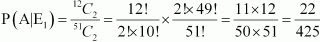When the lost card is not a diamond, there are 13 diamond cards out of 51 cards.
Two cards can be drawn out of 13 diamond cards in 13C2 ways whereas 2 cards can be drawn out of
51 cards in 51C2 ways.
The probability of getting two cards, when one card is lost which is not diamond, is given by P (A|E2).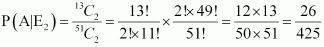The probability that the lost card is diamond is given by P (E1|A).
By using Bayes’ theorem, we obtain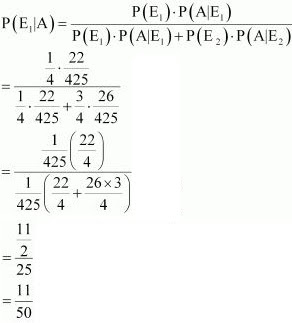13. Probability that A speaks truth is 4/5 . A coin is tossed. A reports that a head appears. The probability that actually there was head is
A. 4/5
B. 1/2
C. 1/5
D. 2/5

Solution

Let E1 and E2 be the events such that
E1: A speaks truth
E2: A speaks false
Let X be the event that a head appears.
P(E1 ) = 45Therefore,
P(E2) = 1-P(E1)=1-45=15If a coin is tossed, then it may result in either head (H) or tail (T).
The probability of getting a head is 1/2 whether A speaks truth or not.
∴ P(X|E1) = P(X|E2 ) = 1/2
The probability that there is actually a head is given by P (E1|X).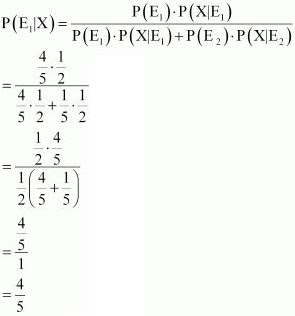Therefore, the correct answer is A.

14. If A and B are two events such that A ⊂ B and P (B) ≠ 0, then which of the following is correct?
(A). P(A|B) = P(B)/P(A)
(B). P(A|B) < P(A)
(C) P(A|B) ≥ P(A)
(D) None of these

Solution

If A ⊂ B, then A ∩ B = A
⇒ P (A ∩ B) = P (A)
Also, P (A) < P (B)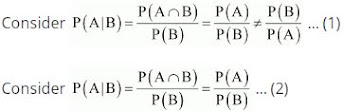It is known that, P(B) ≤ 1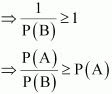From (2), we obtain
⇒ P(A|B) ≥ P(A) ...(3)
∴ P(A|B) is not less than P(A).
Thus, from (3), it can be concluded that the relation given in alternative C is correct.